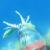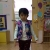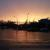## 关于c语言的一个问题

C青苔瓦 发布于 2014/09/15 11:52

00/* 真心话大冒险. v1.0 * */ #include #include #include #include #include #define LINE_MAX 512 #define LINE_NUM_MAX 500 #define WIN 0 #define LINUX 1 FILE *truth_file, *dare_file; char **truth_mem, **dare_mem; int truth_line; int dare_line; int init(void); void game_quit(void); void deal_signal(int); int main(int argc, char **argv) { int quit = 0; int truth_quit = 1; int dare_quit = 1; int main_choice; char truth_choice; char dare_choice; char clear; #if WIN && !LIUNX strncpy(clear, "cls", 10); #elif LINUX && !WIN strncpy(clear, "clear", 10); #endif int randnum; /* 初始化 */ if(!init()) { puts("抱歉!初始化失败."); exit(1); } signal(SIGTERM, deal_signal); /* 终止程序 */ signal(SIGABRT, deal_signal); /* 异常情况 */ /* 主循环 * * */ while(!quit) { puts("0: 退出游戏,如果你才进来,劝你别选!"); puts("1: 真心话哦!你懂的."); puts("2: 大冒险,小心别死了."); puts("只可以输入这三个数字之一,不然,(这里添加颜文字)"); scanf("%d", &main_choice); if(0 == main_choice) quit = 1; else if( 1 == main_choice) { while(0 != truth_quit) { scanf("%c", &truth_choice); if('0' == truth_choice) truth_quit = 0; else if('1' == truth_choice) system(clear); else { randnum = rand() % truth_line; puts(truth_mem[randnum]); } } } else if(2 == main_choice) { while(0 != dare_quit) { scanf("%c", &dare_choice); if('0' == dare_choice) dare_quit = 0; else if('1' == dare_choice) system(clear); else { randnum = rand() % dare_line; puts(dare_mem[randnum]); } } } else { system(clear); puts("Sorry, 你看不懂说明吗??\n\t重来!!!!!\n"); } } /* 退出 */ game_quit(); return 0; } int init(void) { int i; /* 扩展的行是LINE_NUM_MAX的几倍 */ char **ptruth; char **pdare; char line[LINE_MAX]; srand((unsigned int)time(NULL)); /* 初始化随机函数 */ truth_file = fopen("truth_file.dat", "r"); dare_file = fopen("dare_file.dat", "r"); truth_mem = (char **)calloc(LINE_NUM_MAX, sizeof(char *)); dare_mem = (char **)calloc(LINE_NUM_MAX, sizeof(char *)); if(NULL == truth_file || NULL == dare_file) return 0; if(NULL == truth_mem || NULL == dare_mem) { free(truth_mem); free(dare_mem); return 0; } ptruth = truth_mem; pdare = dare_mem; for(truth_line = 0, i = 1; NULL != fgets(line, LINE_MAX, truth_file); truth_line ++) { if(0 < truth_line && 0 == truth_line % LINE_NUM_MAX) { i ++; do { truth_mem = realloc(truth_mem, i * LINE_NUM_MAX); }while(NULL == truth_mem); } do { *ptruth = (char *)malloc(strlen(line) + 1); }while(NULL == *ptruth); strncpy(*ptruth, line, LINE_MAX); ptruth ++; } for(dare_line = 0, i = 1; NULL != fgets(line, LINE_MAX, dare_file); dare_line ++) { if(0 < dare_line && 0 == dare_line % LINE_NUM_MAX) { i ++; do { dare_mem = realloc(dare_mem, i * LINE_NUM_MAX); }while(NULL == dare_mem); } do { *pdare = (char *)malloc(strlen(line) + 1); }while(NULL == *pdare); strncpy(*pdare, line, LINE_MAX); pdare ++; } fclose(truth_file); fclose(dare_file); return 1; } void game_quit(void) { char **ptruth = truth_mem; char **pdare = dare_mem; while(truth_line --) free(*ptruth ++); while(dare_line --) free(*pdare ++); free(truth_mem); free(dare_mem); } void deal_signal(int a) { game_quit(); }
0```/* 真心话大冒险. v1.0 * */
#include <stdlib.h>
#include <stdio.h>
#include <string.h>

#define LINE_MAX 512
#define LINE_NUM_MAX 500
#define WIN 0
#define LINUX 1

FILE *truth_file, *dare_file;
char **truth_mem, **dare_mem;
int truth_line;
int dare_line;

int init(void);

void game_quit(void);

void deal_signal(int);

int main(int argc, char **argv) {
int quit = 0;
int truth_quit = 1;
int dare_quit = 1;
int main_choice;
char truth_choice;
char dare_choice;
char clear;

#if WIN && !LIUNX strncpy(clear, "cls", 10);
#elif LINUX && !WIN strncpy(clear, "clear", 10);
#endif int randnum;
/* 初始化 */
if (!init()) {
puts("抱歉!初始化失败.");
exit(1);
}
signal(SIGTERM, deal_signal); /* 终止程序 */
signal(SIGABRT, deal_signal); /* 异常情况 */
/* 主循环 * * */
while (!quit) {
puts("0: 退出游戏,如果你才进来,劝你别选!");
puts("1: 真心话哦!你懂的.");
puts("2: 大冒险,小心别死了.");
puts("只可以输入这三个数字之一,不然,(这里添加颜文字)");
scanf("%d", &main_choice);
if (0 == main_choice) quit = 1; else if (1 == main_choice) {
while (0 != truth_quit) {
scanf("%c", &truth_choice);
if ('0' == truth_choice) truth_quit = 0; else if ('1' == truth_choice) system(clear); else {
randnum = rand() % truth_line;
puts(truth_mem[randnum]);
}
}
} else if (2 == main_choice) {
while (0 != dare_quit) {
scanf("%c", &dare_choice);
if ('0' == dare_choice) dare_quit = 0; else if ('1' == dare_choice) system(clear); else {
randnum = rand() % dare_line;
puts(dare_mem[randnum]);
}
}
} else {
system(clear);
puts("Sorry, 你看不懂说明吗??\n\t重来!!!!!\n");
}
}
/* 退出 */
game_quit();
return 0;
}

int init(void) {
int i;
/* 扩展的行是LINE_NUM_MAX的几倍 */
char **ptruth;
char **pdare;
char line[LINE_MAX];
srand((unsigned int) time(NULL));
/* 初始化随机函数 */
truth_file = fopen("truth_file.dat", "r");
dare_file = fopen("dare_file.dat", "r");
truth_mem = (char **) calloc(LINE_NUM_MAX, sizeof(char *));
dare_mem = (char **) calloc(LINE_NUM_MAX, sizeof(char *));
if (NULL == truth_file || NULL == dare_file) return 0;
if (NULL == truth_mem || NULL == dare_mem) {
free(truth_mem);
free(dare_mem);
return 0;
}
ptruth = truth_mem;
pdare = dare_mem;
for (truth_line = 0, i = 1; NULL != fgets(line, LINE_MAX, truth_file); truth_line++) {
if (0 < truth_line && 0 == truth_line % LINE_NUM_MAX) {
i++;
do {truth_mem = realloc(truth_mem, i * LINE_NUM_MAX);} while (NULL == truth_mem);
}
do {*ptruth = (char *) malloc(strlen(line) + 1);} while (NULL == *ptruth);
strncpy(*ptruth, line, LINE_MAX);
ptruth++;
}
for (dare_line = 0, i = 1; NULL != fgets(line, LINE_MAX, dare_file); dare_line++) {
if (0 < dare_line && 0 == dare_line % LINE_NUM_MAX) {
i++;
do {dare_mem = realloc(dare_mem, i * LINE_NUM_MAX);} while (NULL == dare_mem);
}
do {*pdare = (char *) malloc(strlen(line) + 1);} while (NULL == *pdare);
strncpy(*pdare, line, LINE_MAX);
pdare++;
}
fclose(truth_file);
fclose(dare_file);
return 1;
}

void game_quit(void) {
char **ptruth = truth_mem;
char **pdare = dare_mem;
while (truth_line--) free(*ptruth++);
while (dare_line--) free(*pdare++);
free(truth_mem);
free(dare_mem);
}

void deal_signal(int a) {
game_quit();
}```

0truth_mem = realloc(truth_mem, i * LINE_NUM_MAX);应该是truth_mem = realloc(truth_mem, i * LINE_NUM_MAX * sizeof(char*));

0do {..malloc()} while(NULL ==p);这种写法，如果内存真得很少了，会不会把系统耗死？分配不到内存直接报错退出就好了。